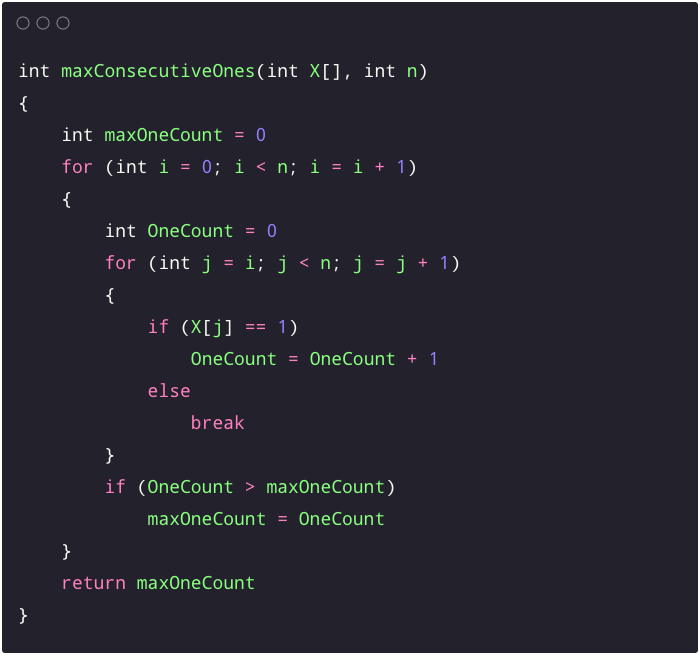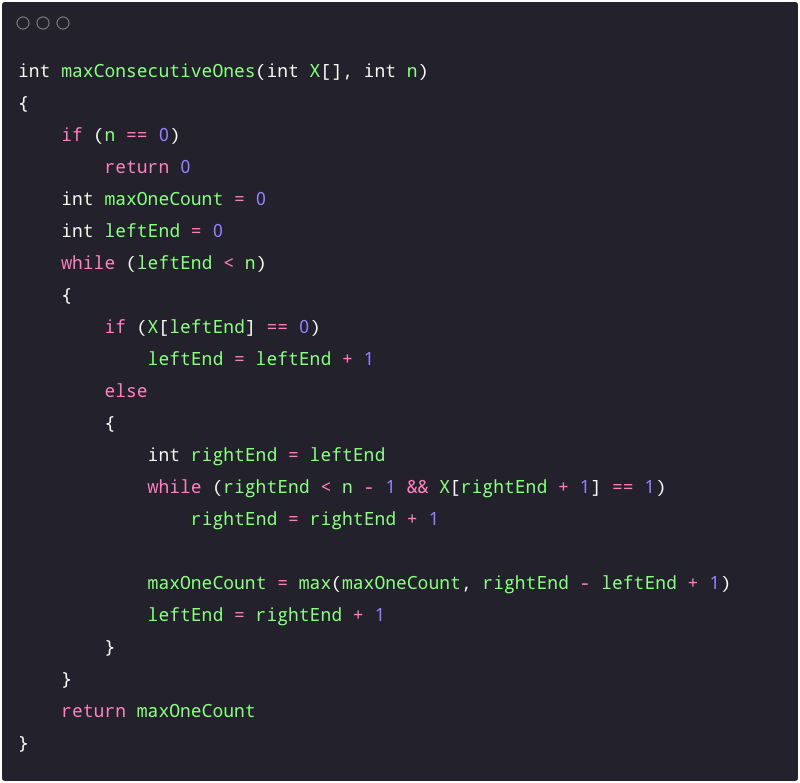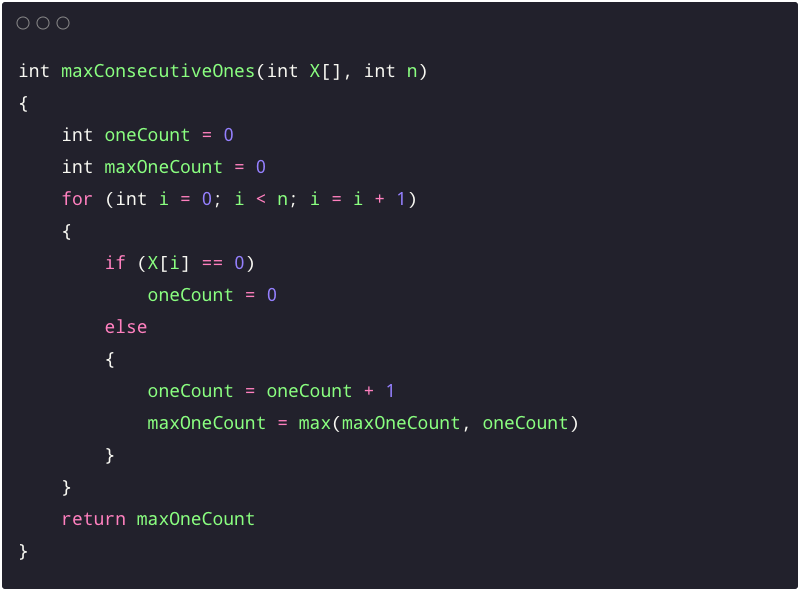# Find Max Consecutive 1's in an Array

Key takeaway: an excellent problem to learn problem-solving using the sliding window approach and an incremental approach using a single loop.

### Let’s understand the problem

An input array X[] is given where all the elements are either 0 or 1. Write a program to find the maximum number of consecutive 1's in the array.

Example 1

Input: X[] = [1, 1, 0, 1, 1, 1, 0, 0, 1], Output: 3

Explanation: There are 6 ones in the array: two consecutive 1's from index 0 to 1, three 1's are present from index 3 to 5, and one 1 is present at index 8. So the max consecutive 1's count is 3.

Example 2

Input: X[] = [0, 1, 1, 1, 1, 0, 0, 1, 1], Output: 4

Explanation: There are 6 ones in the array: four 1's are occurring consecutively from index 1 to 5 and two 1's are present sequentially from index 7 to 8. So the max consecutive 1's count is 4.

Example 3

Input: X[] = [1, 1, 1, 1], Output: 4

Explanation: All values in the array are 1. So the max consecutive 1's count is 4.

Example 4

Input: X[] = [0, 0, 1, 0, 1], Output: 1

Explanation: There is only 1 one in the array. So the max consecutive 1's count is 1.

Important note: Before moving on to the solutions, we recommend trying this problem on paper for atleast 15 or 30 minutes. Enjoy problem-solving!

### Discussed solution approaches

• A brute force solution  by considering every sub-array
• An optimized solution using the sliding window approach
• An efficient solution using a single scan

### A brute force solution  by considering every sub-array

Solution idea

The subarray with max continuous 1's must be starting from some index i and ending at some index j. So one basic idea would be to explore each subarray [i, j] and track the max 1's count. For this, we can run two nested loops: the outer loop to track the starting index (i) of each subarray and the inner loop to calculate consecutive 1's count starting from index i. Using a variable, we also track the overall max 1's count seen so far.

Solution steps

• We initialize the variable maxOneCount = 0 to track max one count so far.
• We run nested loops to explore each subarray i.e. outer loop from i = 0 to n - 1 and inner loop from j = i to n - 1. Before starting the inner loop, we also initialize variable oneCount = 0 to track the ones count in the subarray.
• Inside the inner loop, when X[j] == 1, we increment oneCount by 1. Otherwise, we will be present at the end of 1's sequence, so we break the inner loop.
• Before moving to the next iteration of the outer loop, we compare maxOneCount with oneCount. If maxOneCount < oneCount, we update maxOneCount = oneCount.
• Similarly, we continue exploring all sub-array starting from index i and updating maxOneCount. By the end of the nested loops, we return maxOneCount.

Solution pseudocodeSolution analysis

We are running nested loops to explore each subarray. Total number of loop iterations in the worst case = n + n - 1 + ...+ 2 + 1 = n(n + 1)/2 = O(n^2). At each iteration, we are performing constant or O(1) operations. So time complexity = O(n^2) * O(1) = O(n^2). What would be the scenario of the worst and best case input? Think! Space complexity = O(1), as we are using a constant extra space for variables.

### An optimized solution using the sliding window approach

Solution idea

Now the critical question is: can we improve the time complexity further? Do we need to consider each subarray? If we observe the input closely, we can identify one thing: There will be many groups of consecutive 1's, and we need to identify a group with max consecutive 1's. So rather than exploring every subarray, can we think of an efficient idea to track a group of 1's with the maximum one count?

One idea would be to track the left and right ends of each subarray with consecutive 1's, i.e., the left end tracks the first occurrence of 1, and the right end tracks the last occurrence of 1's in each group. We also need to update the max 1's count found so far. Think!

This solution idea is a sliding window approach, where we move the left end of the window to the first occurrence of 1 in each group and increment the right end until we find 1 in that group. Once the right end reaches the last 1 of the group, we calculate the 1's count and update the max one count so far. Think!

Solution steps

1. We initialize a window pointer leftEnd = 0 to track the left end of the window.
2. We also initialize a variable maxOneCount to track max continuous 1's so far.
3. Now we run a loop till leftEnd < n.
4. We first move leftEnd to the first occurrence of 1. If (X[leftEnd] == 0), we keep incrementing the leftEnd by 1. After this process, we will be at the starting end of the first group.
5. Now we initialize another window pointer rightEnd with leftEnd and run another loop until we find the next zero in the sequence. In this loop, we continue incrementing rightEnd by 1 when we find rightEnd < n - 1 and X[rightEnd + 1] == 1.
6. By the end of the above loop, the window pointer rightEnd will be at the right end of the first group. So total number of consecutive 1's in that group = rightEnd - leftEnd + 1.
7. Now we update the value of maxOneCount: if(maxOneCount < rightEnd - leftEnd + 1), we update maxOneCount with rightEnd - leftEnd + 1. Before moving to the next iteration of the outer loop, we also update the leftEnd with rightEnd + 1 to search the next group of consecutive 1's.
8. Now we follow a similar procedure in all iterations of the outer loop: track the first and last occurrence of 1 in each group using window pointers, and update the value of maxOneCount.
9. By the end of the nested loops, we return maxOneCount.

Solution pseudocodeSolution analysis

We are running two nested loops based on different conditions. Does this time complexity look O(n^2)? Let's analyze it clearly to get an answer.

At each iteration of the outer loop, whenever we find 0, we increment the left pointer. But when we see 1 first time in a group, we continuously increment the right pointer till we find the next 0. At this point, we update the max 1's count and reset the left pointer. So we are accessing each element at once using the left and right pointers. In other words, all the 0's will be accessed by the left pointer, and all the 1's will be accessed by the right pointer.

The total number of nested loop iterations will be O(n), and at each iteration, we perform O(1) operations. So time complexity = O(n) * O(1) = O(n). We are using constant extra space, so space complexity = O(1).

### An efficient solution using a single scan

Solution idea and steps

The critical question is: can we solve the problem using a single scan without using two window pointers? Here is a simple idea: We scan the array from left to right and track the 1's count in each consecutive group of 1's. We also initialize two variables outside the loop: oneCount to store the 1's count of the current group and maxOneCount to store the max 1's count seen so far.

• When we find X[i] == 1, we increment the oneCount by 1 and compare it with the maxOneCount. If (oneCount > maxOneCount), we update maxOneCount = oneCount .
• When we encounter X[i] == 0, we reset the value of oneCount as 0.
• By the end of the loop, we return the value stored in variable maxConseOneCount.

Solution pseudocodeSolution analysis

We are running a single loop and performing constant operations at each iteration. So time complexity = O(n). Space complexity = O(1), as we are using constant extra space.

Important note: we recommend transforming the above pseudo-codes into a favorite programming language (C, C++, Java, Python, etc.) and verifying all the test cases. Enjoy programming!

### Critical ideas to think!

• Can we solve this problem using other approaches like recursion or bit manipulation?
• What would be the worst and best case scenario of the 1st approach?
• How the time complexity of the 2nd approach is O(n)?
• Suppose there are three repeated elements in the array: 0, 1, and 2. How do we modify the above code to count the number of 1's? Can we solve this problem in O(n) time?

### Comparison of time and space complexities

• Considering each sub-array: Time = O(n^2), Space = O(1)
• Using sliding window: Time = O(n^2), Space = O(1)
• Using a single scan: Time = O(n^2), Space = O(1)

### Suggested coding problems to practice

Write in the message below if you find anything incorrect, or you want to share more insight, or you know some different approaches to solve this problem. Enjoy learning, Enjoy algorithms, Enjoy coding!